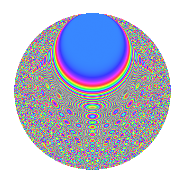# Properties

 Label 1089.2.mLevel 1089 Weight 2 Character orbit m Rep. character $$\chi_{1089}(100,\cdot)$$ Character field $$\Q(\zeta_{11})$$ Dimension 540 Sturm bound 264

# Related objects

## Defining parameters

 Level: $$N$$ = $$1089 = 3^{2} \cdot 11^{2}$$ Weight: $$k$$ = $$2$$ Character orbit: $$[\chi]$$ = 1089.m (of order $$11$$ and degree $$10$$) Character conductor: $$\operatorname{cond}(\chi)$$ = $$121$$ Character field: $$\Q(\zeta_{11})$$ Sturm bound: $$264$$

## Dimensions

The following table gives the dimensions of various subspaces of $$M_{2}(1089, [\chi])$$.

Total New Old
Modular forms 1360 560 800
Cusp forms 1280 540 740
Eisenstein series 80 20 60

## Trace form

 $$540q + 10q^{2} - 60q^{4} + 11q^{5} - 9q^{7} + 8q^{8} + O(q^{10})$$ $$540q + 10q^{2} - 60q^{4} + 11q^{5} - 9q^{7} + 8q^{8} + 7q^{10} + 2q^{11} + 2q^{13} - 7q^{14} - 58q^{16} + 7q^{17} + q^{19} + 15q^{20} + 32q^{22} - 5q^{23} - 81q^{25} - 5q^{26} - 7q^{28} + 5q^{29} - q^{31} + 24q^{32} - 9q^{34} + q^{35} - 40q^{37} + 85q^{38} - 70q^{40} + q^{41} - 17q^{43} + q^{44} - 13q^{46} + 27q^{47} - 15q^{49} - 114q^{50} + 129q^{52} - 3q^{53} + 70q^{55} - 80q^{56} + 30q^{58} + 3q^{59} - 17q^{61} - 66q^{62} - 92q^{64} - 14q^{65} - 68q^{67} + 9q^{68} + 69q^{70} - 41q^{71} + 25q^{73} + 11q^{74} - 243q^{76} + 145q^{77} + 23q^{79} + 188q^{80} + q^{82} + 17q^{83} - 107q^{85} + 15q^{86} - 123q^{88} + 28q^{89} - 94q^{91} + 21q^{92} - 206q^{94} + 99q^{95} - 40q^{97} + 26q^{98} + O(q^{100})$$

## Decomposition of $$S_{2}^{\mathrm{new}}(1089, [\chi])$$ into newform subspaces

The newforms in this space have not yet been added to the LMFDB.

## Decomposition of $$S_{2}^{\mathrm{old}}(1089, [\chi])$$ into lower level spaces

$$S_{2}^{\mathrm{old}}(1089, [\chi]) \cong$$ $$S_{2}^{\mathrm{new}}(121, [\chi])$$$$^{\oplus 3}$$$$\oplus$$$$S_{2}^{\mathrm{new}}(363, [\chi])$$$$^{\oplus 2}$$

## Hecke Characteristic Polynomials

There are no characteristic polynomials of Hecke operators in the database